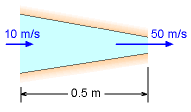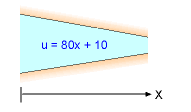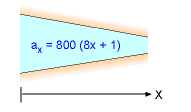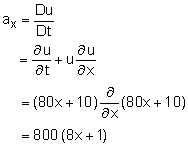Ch 3. Fluid Kinematics Multimedia Engineering Fluids FlowDescriptions Steady &Unsteady Streamlines,Streaklines Velocity &Acceleration IrrotationalFlow
 Chapter 1. Basics 2. Fluid Statics 3. Kinematics 4. Laws (Integral) 5. Laws (Diff.) 6. Modeling/Similitude 7. Inviscid 8. Viscous 9. External Flow 10. Open-Channel Appendix Basic Math Units Basic Equations Water/Air Tables Sections Search eBooks Dynamics Fluids Math Mechanics Statics Thermodynamics Author(s): Chean Chin Ngo Kurt Gramoll ©Kurt GramollFLUID MECHANICS - CASE STUDY SOLUTIONProblem DescriptionVelocity DistributionAcceleration Distribution For the center streamline, the velocity of the fluid is one-dimensional and linear:      u = ax + b where a and b are constants. Based on the experimental measurements, u is 10 m/s when x is zero (inlet) while u is 50 m/s when x is 0.5 m (outlet). Hence, it can be determined that the constants a and b are 80 and 10, respectively. The velocity distribution is thus given by      u = (80x + 10) m/s The acceleration of the fluid is given by (use the fact that v = w = 0 for 1-D flow):The local accelerations of the fluid at the inlet and outlet are then determined to be 800 m/s2 and 4,000 m/s2, respectively.

Practice Homework and Test problems now available in the 'Eng Fluids' mobile app
Includes over 250 problems with complete detailed solutions.
Available now at the Google Play Store and Apple App Store.